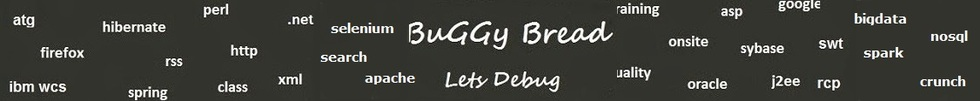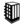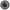Interview Questions and Answers for '1to1help.net' | Search Interview Question - javasearch.buggybread.com# Search Interview QuestionsMore than 3000 questions in repository.There are more than 900 unanswered questions.Have a video suggestion.
Click Correct / Improve and please let us know.
Label / Company      Label / Company / TextSubmit Question

Interview Questions and Answers for '1to1help.net' - 2 question(s) found - Order By NewestQ1. Find Fibonacci first n numbers using recursion ? Core Java
Ans. public int fibonacci(int n) {
if(n == 0)
return 0;
else
if(n == 1)
return 1;
else
return fibonacci(n - 1) fibonacci(n - 2);
}Help us improve. Please let us know the company, where you were asked this question :LikeDiscussCorrect / ImproveAsked in 1 CompaniesRelated QuestionsWhat will happen if we don't have termination statement in recursion ?Write a Program to print factorial of a number using recursionWrite an Algorithm for Graph Traversal ? The Graph has a loop.Write a program to print sum of numbers between the start and end number

For example - Passing start number as 2 and end number as 10, it should print 2+3+4+5+6+7+8+9+10 = 54Write a program using Recursion to print multiplication of numbers between the start and end number

For example - Passing start number as 2 and end number as 10, it should print 2*3*4*5*6*7*8*9*10 = 3628800What is recursion in Java ?What will happen if we don't have termination statement in recursion ?Which of the two - iteration or recursion - is slower ?Write a Program to print fibonacci series using recursion.Q2. What is ViewResolver in Spring MVC ? Spring
Ans. View resolver is used to get view name from Controller and resolve the next view pageHelp us improve. Please let us know the company, where you were asked this question :LikeDiscussCorrect / ImproveAsked in 1 CompaniesRelated QuestionsDifference between == and .equals() ?Why is String immutable in Java ?Explain the scenerios to choose between String , StringBuilder and StringBuffer ?

or

What is the difference between String , StringBuilder and StringBuffer ?What are the difference between composition and inheritance in Java?What is a Lambda Expression ? What's its use ?Explain OOPs

or

Explain OOPs Principles

or

Explain OOPs Concepts

or

Explain OOPs features

orDoes garbage collection guarantee that a program will not run out of memory?Why Char array is preferred over String for storing password?What are different ways to create String Object? Explain.What is the difference between final, finally and finalize() ?